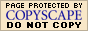"Mixture" Word Problems: Examples (page 2 of 2)

Usually, these exercises are fairly easy to solve once you've found the equations. To help you see how to set up these problems, below are a few more problems with their grids (but not solutions).

• How many liters of a 70% alcohol solution must be added to 50 liters of a 40% alcohol solution to produce a 50% alcohol solution?

 liters sol'n % alcohol total liters alcohol 70% sol'n x 0.70 0.70x 40% sol'n 50 0.40 (0.40)(50) = 20 50% mix 50 + x 0.50 0.50(50 + x)

From the last column, you get the equation 0.7x + 20 = 0.5(50 + x). Solve for x.

• How many ounces of pure water must be added to 50 ounces of a 15% saline solution to make a saline solution that is 10% salt?

 ounces liquid % salt total ounces salt water x 0 0 15% sol'n 50 0.15 (50)(0.15) = 7.5 10% mix 50 + x 0.10 0.10(50 + x)

From the last column, you get the equation 7.5 = 0.1(50 + x). Solve for x.

(Note the percentage for water. "Pure water" contains no salt, so the percent of salt is zero. If, on the other hand, you were trying to increase the salt content by adding pure salt, the percent would have been one hundred.)

• Find the selling price per pound of a coffee mixture made from 8 pounds of coffee that sells for \$9.20 per pound and 12 pounds of coffee that costs \$5.50 per pound.

 pounds coffee \$/pound total \$ for coffee pricey 8 \$9.20 (8)(\$9.20) = \$73.60 cheapo 12 \$5.50 (12)(\$5.50) = \$66 mix 8 + 12 = 20 ? \$73.60 + 66 = \$139.60

From the last row, you see that you have 20 pounds for \$139.60, or \$139.60/(20 pounds). Simplify the division to find the unit rate.   Copyright © Elizabeth Stapel 1999-2011 All Rights Reserved

• How many pounds of lima beans that cost \$0.90 per pound must be mixed with 16 pounds of corn that costs \$0.50 per pound to make a mixture of vegetables that costs \$0.65 per pound?

 pounds \$/pound total \$ for veggies lima beans x \$0.90 \$0.90x corn 16 \$0.50 (16)(\$0.50) = \$8 mix 16 + x \$0.65 (16 + x)(\$0.65)

From the last column, you get the equation \$0.90x + \$8 = (16 + x)(\$0.65). Solve for x.

• Two hundred liters of a punch that contains 35% fruit juice is mixed with 300 liters (L) of another punch. The resulting fruit punch is 20% fruit juice. Find the percent of fruit juice in the 300 liters of punch.

 liters punch % juice total liters juice 35% juice 200 0.35 (200)(0.35) = 70 other punch 300 x 300x mix 200 + 300 = 500 0.20 (500)(0.20) = 100

From the last column, you get the equation 70 + 300x = 100. Solve for x, and then convert the decimal answer to a percentage.

• Ten grams of sugar are added to a 40-g serving of a breakfast cereal that is 30% sugar. What is the percent concentration of sugar in the resulting mixture?

 grams in bowl % sugar total grams sugar sugar 10 1.00 10 cereal 40 0.30 (40)(0.30) = 12 mix 50 ? 10 + 12 = 22

From the last row, you see that there are 22 grams of sugar in the 50 grams in the bowl, or 22/50. Simplify, and then convert to a percentage.